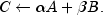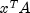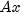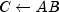JMSLTM Numerical Library 7.2.0
com.imsl.math

## Class ComplexSparseMatrix

• All Implemented Interfaces:
Serializable

```public class ComplexSparseMatrix
extends Object
implements Serializable```
Sparse matrix of type `Complex`. The class represents a general complex sparse matrix. It is intended to be efficiently and easily updated.

A `ComplexSparseMatrix` can be constructed from a set of arrays, or it can be abstractly created as an empty array and then incrementally built into final form. It is usually easier to create an empty `ComplexSparseMatrix` of set size and then use the `set` method to set the elements of the array. When setting the elements of the sparse array, their positions should be thought of as their positions in the dense array. Elements can be set in any order, but only the elements set are stored.

This class includes methods to update the sparse matrix. There are also methods to multiply a sparse matrix and a vector or to multiply two sparse matrices. To solve a sparse linear system use `ComplexSparseCholesky` or `ComplexSuperLU`.

`ComplexSparseCholesky`, `ComplexSuperLU`, Example: Create from Arrays, Serialized Form
• ### Nested Class Summary

Nested Classes
Modifier and Type Class and Description
`static class ` `ComplexSparseMatrix.SparseArray`
The `SparseArray` class uses public fields to hold the data for a sparse matrix in the Java Sparse Array format.
• ### Constructor Summary

Constructors
Constructor and Description
`ComplexSparseMatrix(ComplexSparseMatrix.SparseArray sparseArray)`
Constructs a complex sparse matrix from a `SparseArray` object.
`ComplexSparseMatrix(ComplexSparseMatrix A)`
Creates a new instance of `ComplexSparseMatrix` which is a copy of another `ComplexSparseMatrix`.
```ComplexSparseMatrix(int nRows, int nColumns)```
Creates a new instance of `ComplexSparseMatrix`.
```ComplexSparseMatrix(int nRows, int nColumns, int[][] index, Complex[][] values)```
Constructs a sparse matrix from SparseArray (Java Sparse Array) data.
• ### Method Summary

Methods
Modifier and Type Method and Description
`static ComplexSparseMatrix` ```add(Complex alpha, Complex beta, ComplexSparseMatrix A, ComplexSparseMatrix B)```
Performs element-wise addition of two complex sparse matrices `A`, `B` of type `ComplexSparseMatrix`,`void` `checkSquareMatrix()`
Check that the matrix is square.
`ComplexSparseMatrix` `conjugateTranspose()`
Returns the conjugate transpose of the matrix.
`double` `frobeniusNorm()`
Returns the Frobenius norm of the matrix.
`Complex` ```get(int iRow, int jColumn)```
Returns the value of an element in the matrix.
`int` `getNumberOfColumns()`
Returns the number of columns in the matrix.
`long` `getNumberOfNonZeros()`
Returns the number of nonzeros in the matrix.
`int` `getNumberOfRows()`
Returns the number of rows in the matrix.
`double` `infinityNorm()`
Returns the infinity norm of the matrix.
`Complex[]` `multiply(Complex[] x)`
Multiply the matrix by a vector.
`static Complex[]` ```multiply(Complex[] x, ComplexSparseMatrix A)```
Multiply row array `x` and sparse matrix `A`,.
`static Complex[]` ```multiply(ComplexSparseMatrix A, Complex[] x)```
Multiply sparse matrix `A` and column array `x`,.
`static ComplexSparseMatrix` ```multiply(ComplexSparseMatrix A, ComplexSparseMatrix B)```
Multiply two sparse complex matrices A and B,.
`static Complex[]` ```multiplyHermitian(ComplexSparseMatrix A, Complex[] x)```
Multiply sparse Hermitian matrix `A` and column vector `x`.
`double` `oneNorm()`
Returns the matrix one norm of the sparse matrix.
`Complex` ```plusEquals(int iRow, int jColumn, Complex x)```
Adds a value to an element in the matrix.
`void` ```set(int iRow, int jColumn, Complex x)```
Sets the value of an element in the matrix.
`Complex[][]` `toDenseMatrix()`
Returns the sparse matrix as a dense matrix.
`ComplexSparseMatrix.SparseArray` `toSparseArray()`
Returns the sparse matrix in the `SparseArray` form.
• ### Methods inherited from class java.lang.Object

`clone, equals, finalize, getClass, hashCode, notify, notifyAll, toString, wait, wait, wait`
• ### Constructor Detail

• #### ComplexSparseMatrix

`public ComplexSparseMatrix(ComplexSparseMatrix.SparseArray sparseArray)`
Constructs a complex sparse matrix from a `SparseArray` object.
Parameters:
`sparseArray` - is a `SparseArray` used to initialize the sparse matrix. The field `numberOfNonZeros` in `SparseArray` is not used for initialization, so it does not have to be set.
• #### ComplexSparseMatrix

`public ComplexSparseMatrix(ComplexSparseMatrix A)`
Creates a new instance of `ComplexSparseMatrix` which is a copy of another `ComplexSparseMatrix`.
Parameters:
`A` - is the `ComplexSparseMatrix` to be copied.
• #### ComplexSparseMatrix

```public ComplexSparseMatrix(int nRows,
int nColumns)```
Creates a new instance of `ComplexSparseMatrix`. Initially this is the zero matrix.
Parameters:
`nRows` - an `int` containing the number of rows in the sparse matrix.
`nColumns` - an `int` containing the number of columns in the sparse matrix.
• #### ComplexSparseMatrix

```public ComplexSparseMatrix(int nRows,
int nColumns,
int[][] index,
Complex[][] values)```
Constructs a sparse matrix from SparseArray (Java Sparse Array) data.
Parameters:
`nRows` - an `int` containing the number of rows in the sparse matrix.
`nColumns` - an `int` containing the number of columns in the sparse matrix.
`index` - an `int` jagged array containing the column indices of all nonzero elements corresponding to the compressed representation of the sparse matrix in `values`. The size of `index` must be identical to the size of `values`. The i-th row contains the column indices of all nonzero elements of row i of the sparse matrix. The j-th element of row i is the column index of the value located at the same position in `values`.
`values` - a `Complex` jagged array containing the compressed representation of a complex sparse matrix of size `nRows` by `nColumns`. The number of rows in `values` must be `nRows`. The i-th row contains all nonzero elements of row i of the full sparse matrix.
• ### Method Detail

```public static ComplexSparseMatrix add(Complex alpha,
Complex beta,
ComplexSparseMatrix A,
ComplexSparseMatrix B)```
Performs element-wise addition of two complex sparse matrices `A`, `B` of type `ComplexSparseMatrix`,Parameters:
`alpha` - a `Complex` scalar.
`beta` - a `Complex` scalar.
`A` - a `ComplexSparseMatrix` matrix.
`B` - a `ComplexSparseMatrix` matrix.
Returns:
a `ComplexSparseMatrix` matrix representing the computed sum.
Throws:
`IllegalArgumentException` - This exception is thrown if the matrices are not of the same size.
• #### checkSquareMatrix

`public void checkSquareMatrix()`
Check that the matrix is square.
Throws:
`IllegalArgumentException` - is thrown if the matrix is not square.
• #### conjugateTranspose

`public ComplexSparseMatrix conjugateTranspose()`
Returns the conjugate transpose of the matrix.
Returns:
a `ComplexSparseMatrix` object which is the conjugate transpose of the constructed `ComplexSparseMatrix` object.
• #### frobeniusNorm

`public double frobeniusNorm()`
Returns the Frobenius norm of the matrix.
Returns:
a `double` scalar value equal to the Frobenius norm of the matrix.
• #### get

```public Complex get(int iRow,
int jColumn)```
Returns the value of an element in the matrix.
Parameters:
`iRow` - an `int` containing the row index of the element.
`jColumn` - an `int` containing the column index of the element.
Returns:
a `Complex` containing the value of the `iRow`-th and `jColumn`-th element. If the element was never set, its value is zero.
• #### getNumberOfColumns

`public int getNumberOfColumns()`
Returns the number of columns in the matrix.
Returns:
an `int` containing the number of columns in the matrix.
• #### getNumberOfNonZeros

`public long getNumberOfNonZeros()`
Returns the number of nonzeros in the matrix.
Returns:
a `long` containing the number of nonzeros in the matrix.
• #### getNumberOfRows

`public int getNumberOfRows()`
Returns the number of rows in the matrix.
Returns:
an `int` containing the number of rows in the matrix.
• #### infinityNorm

`public double infinityNorm()`
Returns the infinity norm of the matrix.
Returns:
a `double` scalar value equal to the maximum of the row sums of the absolute values of the array elements of the sparse matrix.
• #### multiply

`public Complex[] multiply(Complex[] x)`
Multiply the matrix by a vector.
Parameters:
`x` - a `Complex` column array.
Returns:
a `Complex` vector representing the product of this matrix times x.
Throws:
`IllegalArgumentException` - This exception is thrown if the number of columns in the `ComplexSparseMatrix` object is not equal to the number of elements in the input column vector.
• #### multiply

```public static Complex[] multiply(Complex[] x,
ComplexSparseMatrix A)```
Multiply row array `x` and sparse matrix `A`,.
Parameters:
`x` - a `Complex` row array.
`A` - a `ComplexSparseMatrix` matrix.
Returns:
a `Complex` vector representing the product of the arguments,.
Throws:
`IllegalArgumentException` - This exception is thrown when the number of elements in the input vector is not equal to the number of rows of the matrix.
• #### multiply

```public static Complex[] multiply(ComplexSparseMatrix A,
Complex[] x)```
Multiply sparse matrix `A` and column array `x`,.
Parameters:
`A` - a `ComplexSparseMatrix` matrix.
`x` - a `Complex` column array.
Returns:
a `Complex` vector representing the product of the arguments,.
Throws:
`IllegalArgumentException` - This exception is thrown when the number of columns in the input matrix is not equal to the number of elements in the input column vector.
• #### multiply

```public static ComplexSparseMatrix multiply(ComplexSparseMatrix A,
ComplexSparseMatrix B)```
Multiply two sparse complex matrices A and B,.
Parameters:
`A` - a `ComplexSparseMatrix` sparse matrix.
`B` - a `ComplexSparseMatrix` sparse matrix.
Returns:
the `ComplexSparseMatrix` product AB of A and B.
Throws:
`IllegalArgumentException` - This exception is thrown when the column number of matrix A is not equal to the row number of matrix B.
• #### multiplyHermitian

```public static Complex[] multiplyHermitian(ComplexSparseMatrix A,
Complex[] x)```
Multiply sparse Hermitian matrix `A` and column vector `x`.
Parameters:
`A` - a `ComplexSparseMatrix` sparse Hermitian matrix, where only the lower triangular part of the matrix is to be used.
`x` - A `Complex` vector.
Returns:
a `Complex` vector representing the product of the arguments,.
Throws:
`IllegalArgumentException` - This exception is thrown when the input matrix is not square or the number of columns in the input matrix is not equal to the number of elements in the input column vector.
• #### oneNorm

`public double oneNorm()`
Returns the matrix one norm of the sparse matrix.
Returns:
a `double` value equal to the maximum of the column sums of the absolute values of the array elements.
• #### plusEquals

```public Complex plusEquals(int iRow,
int jColumn,
Complex x)```
Adds a value to an element in the matrix.
Parameters:
`iRow` - an `int` containing the row index of the element.
`jColumn` - an `int` containing the column index of the element.
`x` - a `Complex` containing the value to be added.
Returns:
a `Complex` containing the updated value of the element, which equals its old value plus x.
• #### set

```public void set(int iRow,
int jColumn,
Complex x)```
Sets the value of an element in the matrix.
Parameters:
`iRow` - an `int` containing the row index of the element.
`jColumn` - an `int` containing the column index of the element.
`x` - a `Complex` containing the value of the `iRow`-th and `jColumn`-th element.
• #### toDenseMatrix

`public Complex[][] toDenseMatrix()`
Returns the sparse matrix as a dense matrix.
Returns:
a rectangular Java array of type `Complex` containing this matrix with all of the zeros explicitly present. The number of rows and columns in the returned matrix is the same as in the sparse matrix.
• #### toSparseArray

`public ComplexSparseMatrix.SparseArray toSparseArray()`
Returns the sparse matrix in the `SparseArray` form.
JMSLTM Numerical Library 7.2.0